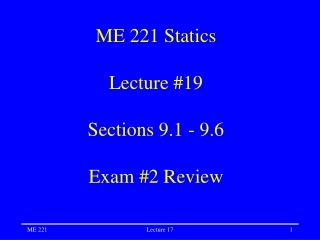# ME 221 Statics Lecture #19 Sections 9.1 - 9.6 Exam #2 Review - PowerPoint PPT PresentationDownload PresentationME 221 Statics Lecture #19 Sections 9.1 - 9.6 Exam #2 Review

Download Presentation## ME 221 Statics Lecture #19 Sections 9.1 - 9.6 Exam #2 Review

- - - - - - - - - - - - - - - - - - - - - - - - - - - E N D - - - - - - - - - - - - - - - - - - - - - - - - - - -
##### Presentation Transcript

1. Homework #6 • Chapter 4 problems: • 2, 4, 10, 11, 18, 24, 39, 42, 43 & 47 • Due Today Lecture 17

2. Homework #7 • Chapter 4 problems: • 52, 53 & 54 • Chapter 9 problems: • 2, 3, 4, 11, 23, 29, 32 • Use double integration for 2, 3, 4 & 11 • Due Friday, October 17 Lecture 17

3. Exam 2 • Friday, October 10 • More on the exam later in the hour Lecture 17

4. x dA y r Moments of Area • Characterizes the way area is distributed about the centroid • Always a positive value with units of in4, mm4, ft4, m4, etc. • Area Moment of Inertia (Second moment of an area) Lecture 17

5. y y y dx dy x x x a dx dx dy Example dA=dxdy dIxx=y2dA dIyy=x2dA dA=(a-x)dy dIxx=y2dA dA=ydx dIyy=x2dA use horizontal element for value in x use vertical element for value in y Lecture 17

6. y x y x Moments of Basic Shapes • Rectangle Common values found in table 9.1, p451 • Circular Lecture 17

7. y r x Polar Moment The polar moment is the second moment of area about the z-axis (resistance to torsion) Note that: Ixx + Iyy = JOz Lecture 17

8. y x’ C d x Parallel Axis Theorem The centroid of the area MUST be one of the axes used in the parallel axis theorem. Lecture 17

9. Radius of Gyration An alternate, equivalent way to represent the moment of an area Distance to where the area is concentrated Lecture 17

10. Example Lecture 17

11. ME 221 Exam 2 Topics • Chapter 3 • Calculating moments • Cross products • Moment of a force about an axis • Moment of a couple • Equivalent force systems Lecture 17

12. ME 221 Exam 2 Topics • Chapter 4 • Center of mass • Centroids of lines, areas and volumes • Centroids of composite bodies • Distributed loads on beams Lecture 17